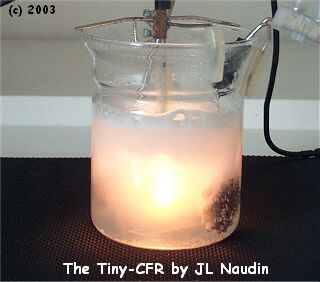Tests of a Tiny Cold Fusion Reactor
created on May 8, 2003 - JLN Labs - Last update May 18, 2003
Toutes les informations et schémas sont publiés gratuitement ( freeware ) et sont destinés à un usage personnel et non commercial
All informations and diagrams are published freely (freeware) and are intended for a private use and a non commercial use.

The Cold Fusion Reactor ( CFR ) is fully based on the work of the researchers Tadahiko MIZUNO and Tadayoshi OHMORI from the Hokkaido University in Japan.

1 - Cold Fusion Reactor ( Tiny-CFR ) - Description :

The Tiny Cold Fusion Reactor is composed of a small pyrex beaker filled with a 150 mL solution of Sodium Hydrogenocarbonate ( NaHCO3 ) 0.5 molar ( 0.5 M ). The Sodium Hydrogenocarbonate ( NaHCO3 ) or baking soda is commonly named in France " Bicarbonate de Soude ".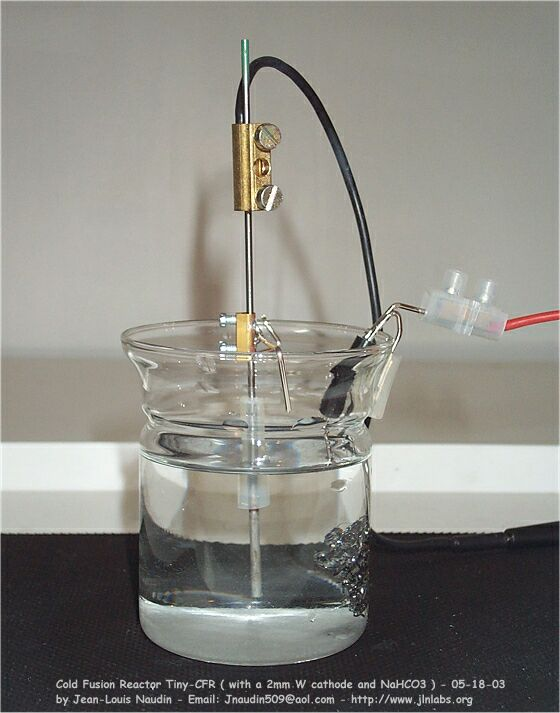The Cathode used is a pure tungsten rod ( W ) 2 mm diameter and 25 mm length from tungsten welding rods. The Anode used is composed of stainless steel mesh maintained with a stainless steel  shaft. All the wires connections are made with a 1.5 mm2 copper flexible wire gained with silicon.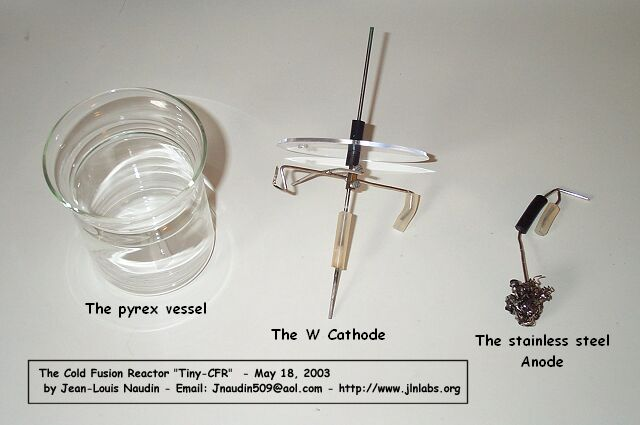The W-Cathode is a 2mm diameter and 150 mm tungsten welding rod.

2 - Experimental test setup :

Three tests have been conducted on the Tiny-CFR, one test at LOW POWER and two tests at a MEDIUM POWER. In all the cases the voltage have been set so as to get a glowing plasma around the cathode.

2-1 - TEST #1 AT A LOW POWER :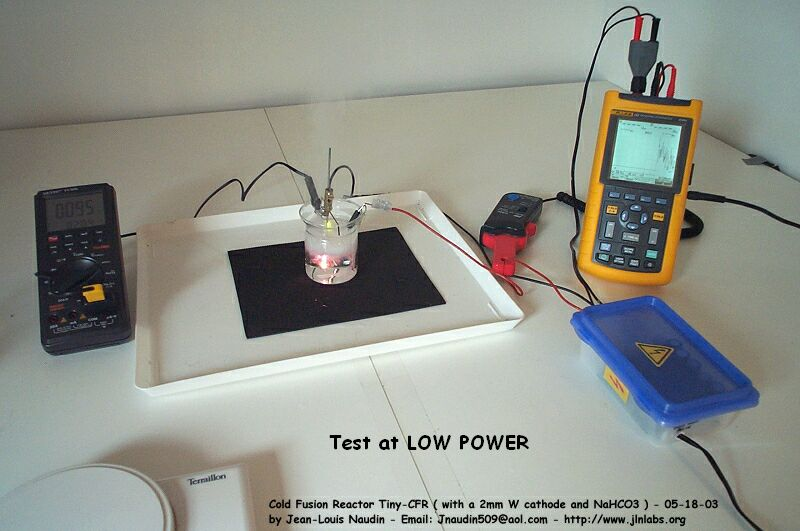The Tiny-CFR is powered with a DC voltage through a bridge rectifier connected to an isolation transformer. The voltage input has been measured with a digital oscilloscope Fluke 123 with a Shielded Test Lead STL 120 ( 1:1, 1 Mohms/225 pF ). The current input has been measured with a current clamp CIE Model CA-60A ( Accuracy DC Amps ±1.5%, AC Amps±2% (40Hz-2kHz), AC Amps±4%(2kHz-10kHz), AC Amps±6%(10kHz-20kHz) ). The temperature has been measured with a type "K" temp probe ( NiCrNi ) connected on a VC506 digital multimeter ( -20°C to +1200 °C with an accuracy of +/- 3% ). ( see the photo above ).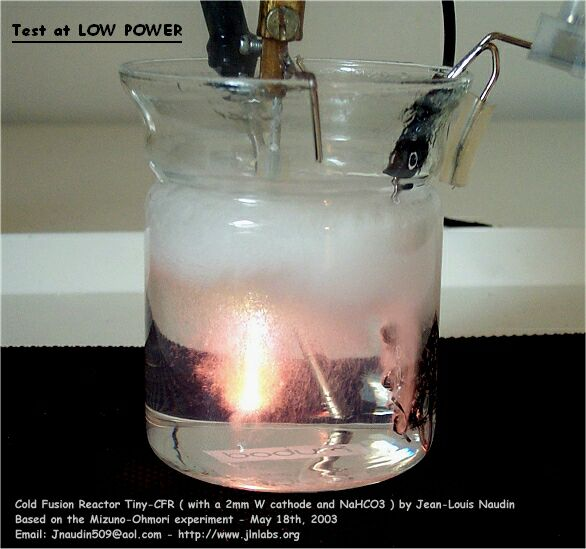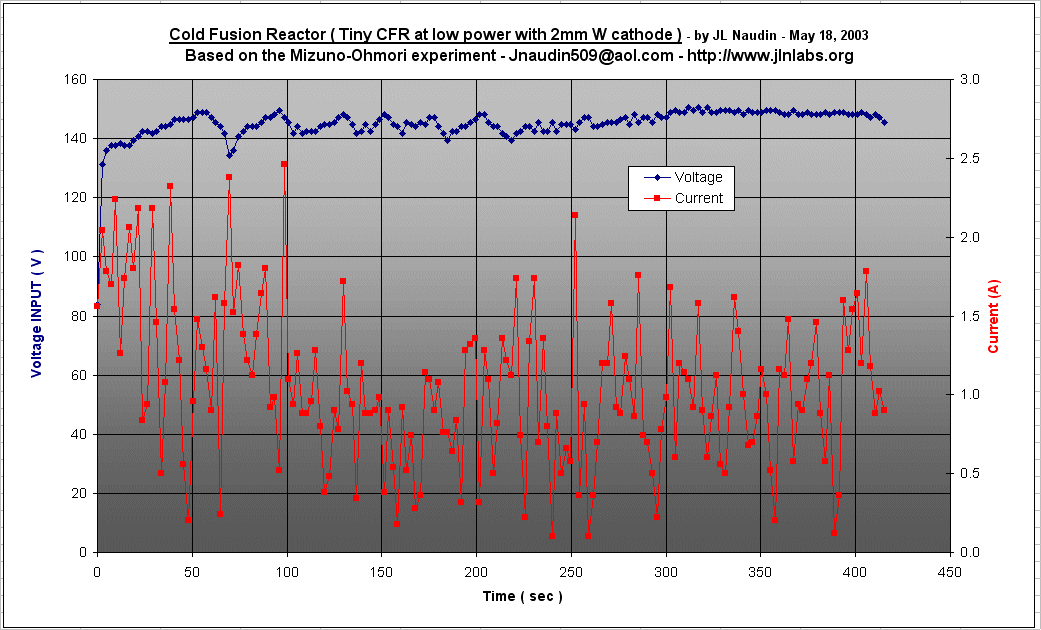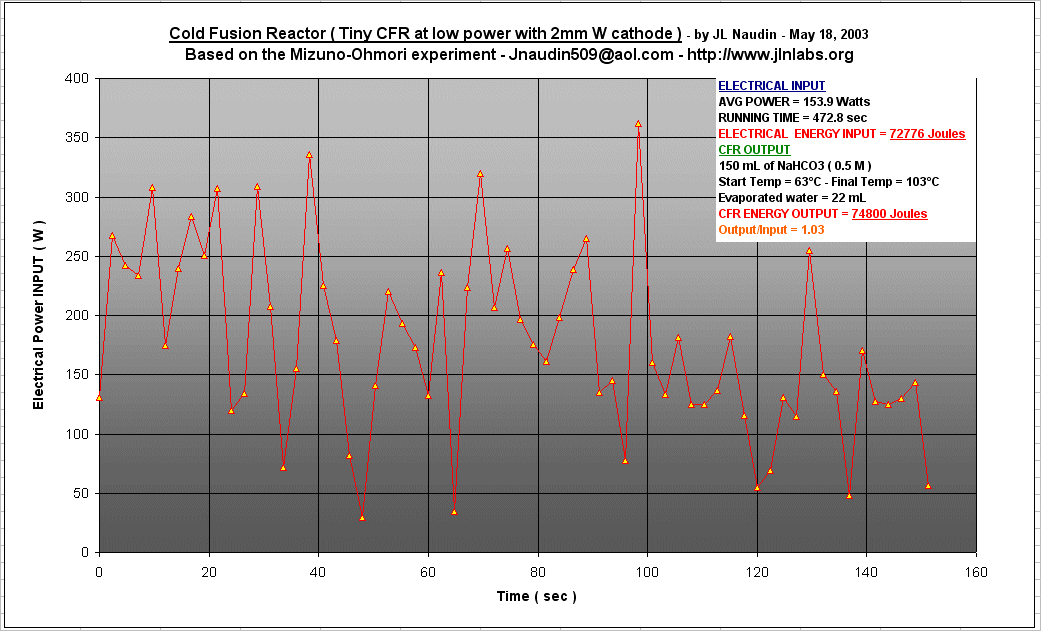POWER INPUT/OUTPUT - Low Power TEST RESULTS :

The Voltage/Current datas logged give an average electrical power input of 153.9 Watts during 472.8 seconds, so this gives :

ELECTRICAL ENERGY INPUT = 72776 Joules

The evaporated water in the CFR during the full boiling was 22 mL. We know that we need 2260 J/g to vaporize water. The temperature rise of the 150 mL was 40°C. So, this gives :

ENERGY OUTPUT = ( 22 x 2260 ) + ( 150 x 40 x 4.18 ) = 74800 Joules

Power OUTPUT = 158.2 Watts, Power Gain = 4.3 Watts

 Energy OUTPUT/INPUT = 74800 / 72776 = 1.03

2-2 - TEST #2 AT A MEDIUM POWER :

TheTiny-CFR has been equiped with a plastic disk placed above the pyrex beaker for avoiding that some water be lost during the strong reaction inside the reactor .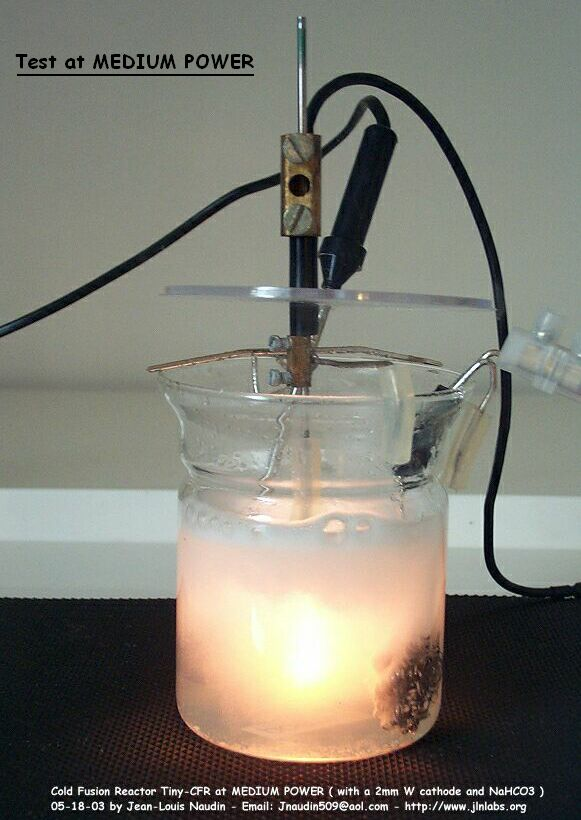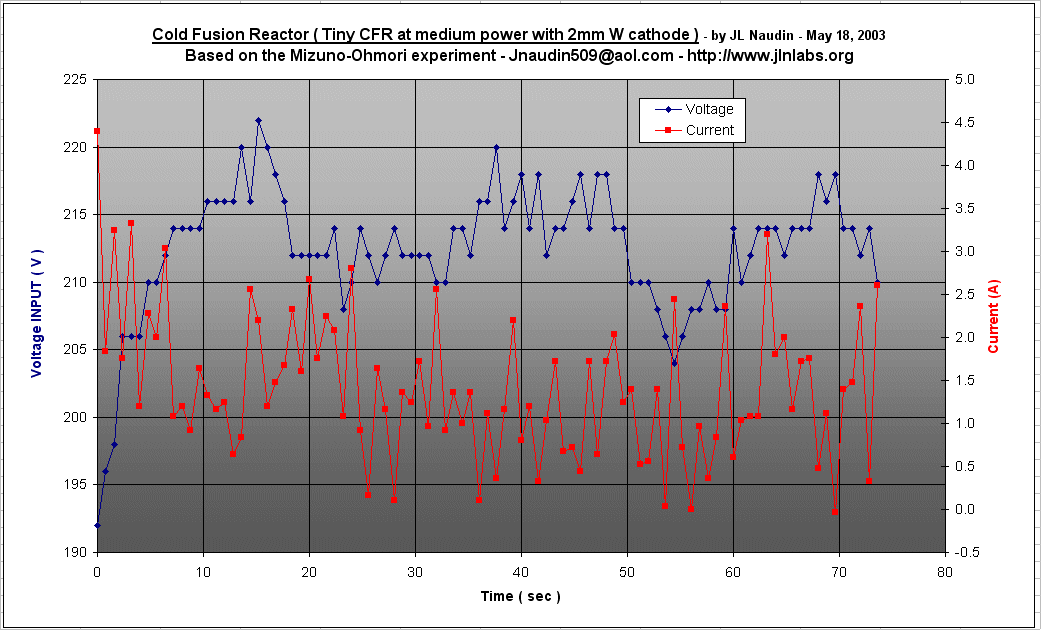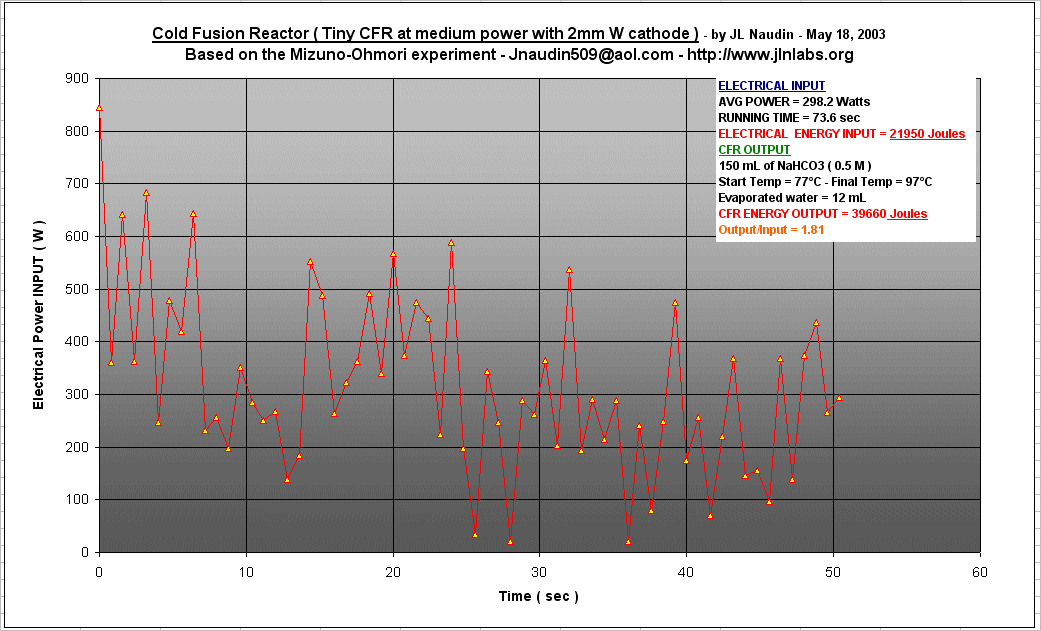POWER INPUT/OUTPUT - Medium Power TEST RESULTS :

The Voltage/Current datas logged give an average electrical power input of 298.2 Watts during 73.6 seconds, so this gives :

ELECTRICAL ENERGY INPUT = 21950 Joules

The evaporated water in the CFR during the full boiling was 12 mL. We know that we need 2260 J/g to vaporize water. The temperature rise of the 150 mL was 20°C. So, this gives :

ENERGY OUTPUT = ( 12 x 2260 ) + ( 150 x 20 x 4.18 ) = 39660 Joules

Power OUTPUT = 538.9 Watts, Power Gain = 240.7 Watts

 Energy OUTPUT/INPUT = 39660 / 21950 = 1.81

2-3 - TEST #3 AT A MEDIUM POWER :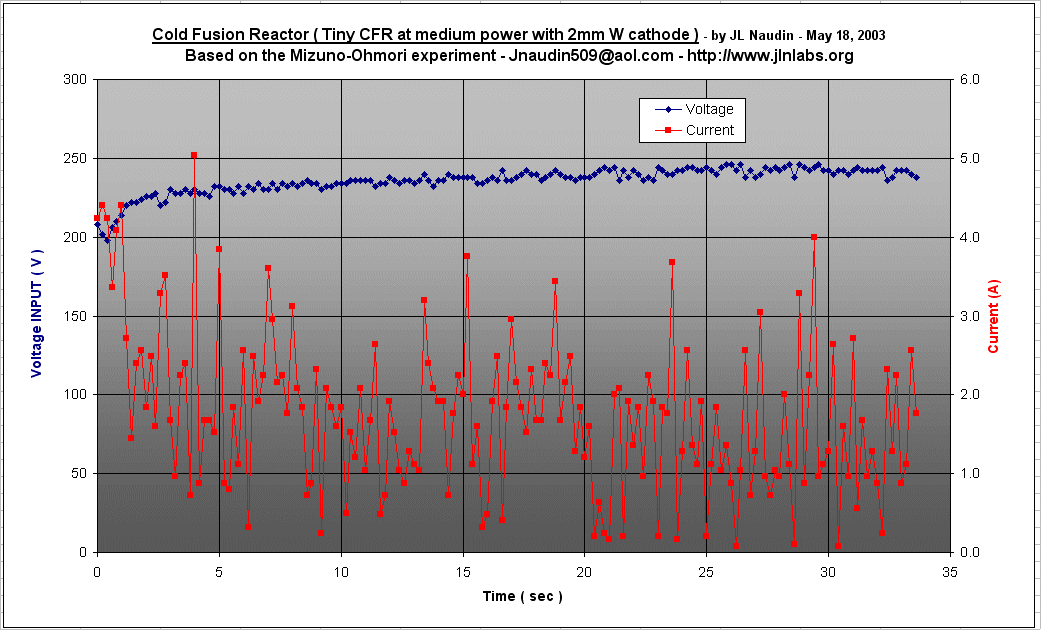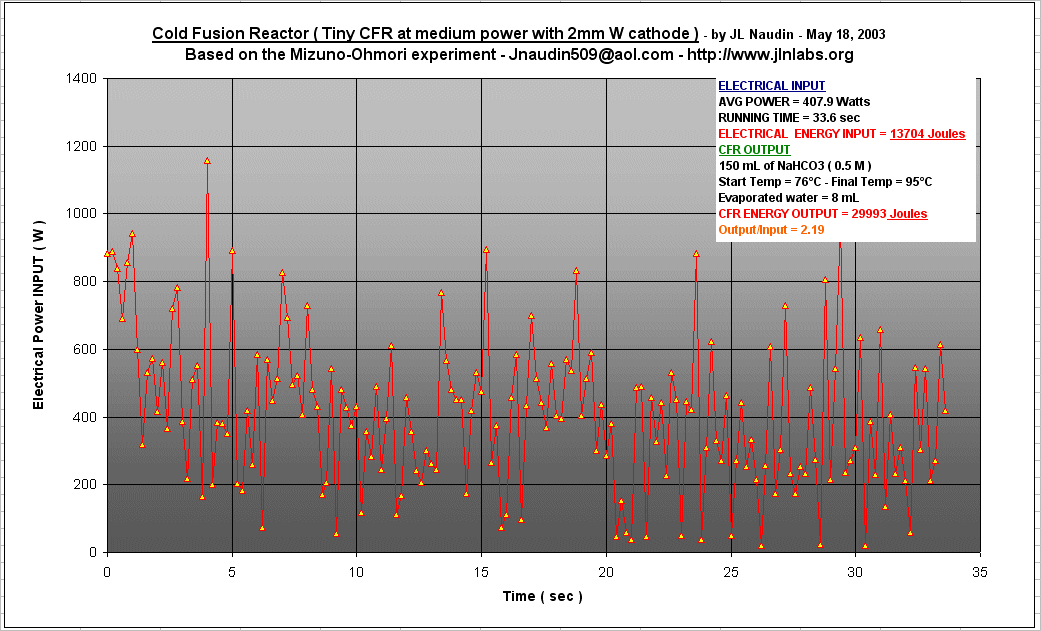POWER INPUT/OUTPUT - Medium Power TEST RESULTS :

The Voltage/Current datas logged give an average electrical power input of 407.9 Watts during 33.6 seconds, so this gives :

ELECTRICAL ENERGY INPUT = 13704 Joules

The evaporated water in the CFR during the full boiling was 8 mL. We know that we need 2260 J/g to vaporize water. The temperature rise of the 150 mL was 19°C. So, this gives :

ENERGY OUTPUT = ( 8 x 2260 ) + ( 150 x 19 x 4.18 ) = 29993 Joules

Power OUTPUT = 892.6 Watts, Power Gain = 484.7 Watts

 Energy OUTPUT/INPUT = 29993 / 13704 = 2.19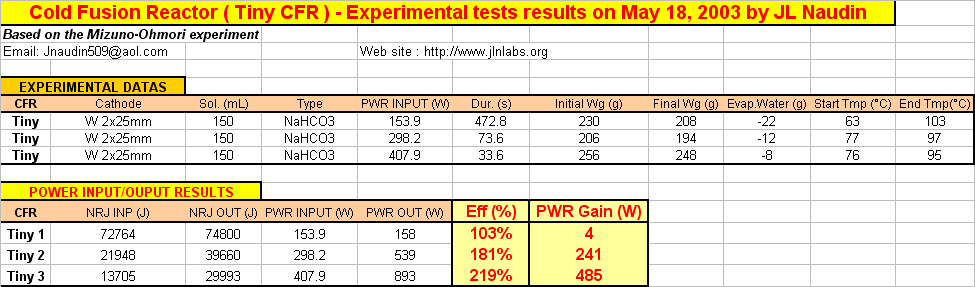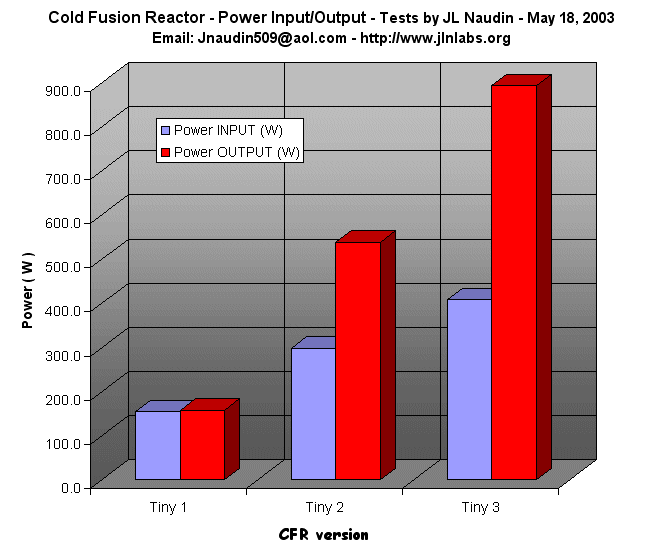See a video of full demonstration of the Tiny-CFR in action

To see the video, the free downloadable RealPlayer is required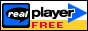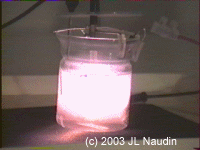Animated video of the Tiny-CFR in action

Interesting documents and references :

See the previous tests :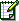Email : JNaudin509@aol.com